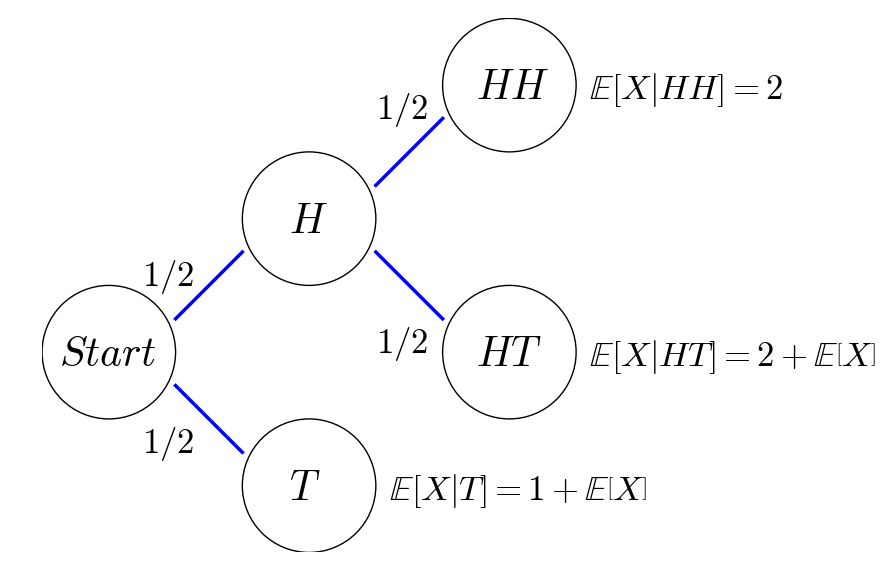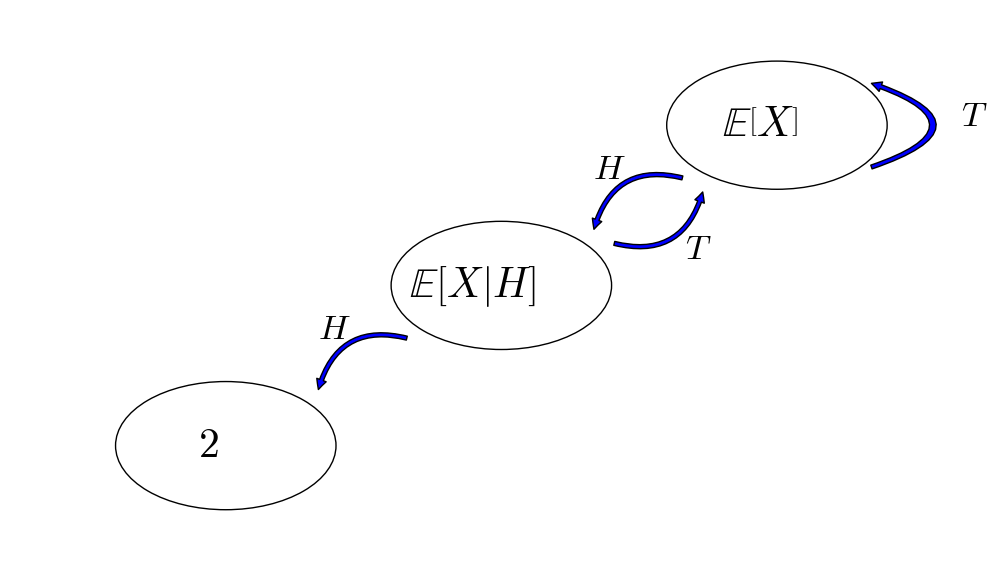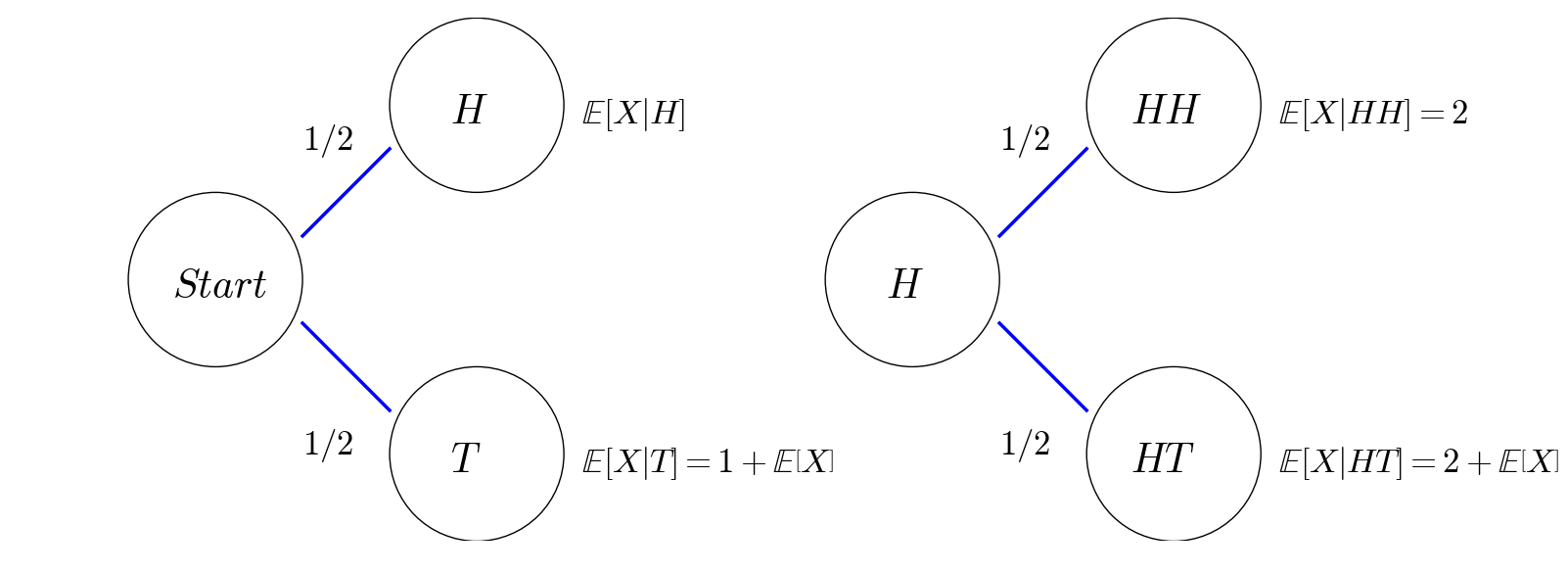Source

## Problem

Suppose we toss a fair coin, what is the expected number of tosses until we get two heads in a row?

I’ll show three ways of solving this problem.

## Method I: Recursive Expectation in Two Steps

Let $X$ be the number of tosses until we get two heads in a row, then we use the recursive relations of expectations to write down an equation of $\mathbb{E}\left[X\right]$ as follows:

This recursive relation can be better explained by the probabilistic tree below:For those who know Markov chains, we can also draw a state transition diagram as follows:## Method II: Recursive Expectation in One Step

This mehod is almost the same as Method I, except that we use the recursive relations of expections to write down a system of equations to solve:As you see more examples in my next post, it is more common to formulate a system of equation, rather than a single equation, for the problem of recursive expectations.

## Method III: Martingale Approach (Optional)

We introduce a random process $X_n,n\geq 1$ as a random process to represent the coin toss process, that is,

Note that $X_n$ is a martingale. And we use another random process $Y_n,n\geq 1$ to represent our trading strategy,

This means that we start our first bet with $1$, then we double our bet to $2$ if the previous coit toss turns out to be head and still bet $1$ otherwise.

Consequently, our wealth process $Z_n = Y_1X_1 + \sum_{i=2}^n Y_i(X_i - X_{i-1})$ is given by

Indeed, $Z_n$ is a discrete-time stochastic integral with respect to $X_n$, and therefore, $Z_n$ is also a martingale.

Now, we define a stopping time $\tau = \min\{n\geq 2: X_n = 1, X_{n-1}=1\}$. The definition of $\tau$ would imply that $X_1=\cdots=X_{\tau-2}=0,X_{\tau-1}=X_{\tau}=1$.

Finally, we invoke the optinal sampling theorem to get

Written on August 2, 2017